# Visualize Image Classifications Using Maximal and Minimal Activating Images

This example shows how to use a data set to find out what activates the channels of a deep neural network. This allows you to understand how a neural network works and diagnose potential issues with a training data set.

This example covers a number of simple visualization techniques, using a GoogLeNet transfer-learned on a food data set. By looking at images that maximally or minimally activate the classifier, you can discover why a neural network gets classifications wrong.

### Load and Preprocess the Data

Load the images as an image datastore. This small data set contains a total of 978 observations with 9 classes of food.

Split this data into a training, validation, and test sets to prepare for transfer learning using GoogLeNet. Display a selection of images from the data set.

```rng default dataDir = fullfile(tempdir,"Food Dataset"); url = "https://www.mathworks.com/supportfiles/nnet/data/ExampleFoodImageDataset.zip"; if ~exist(dataDir,"dir") mkdir(dataDir); end downloadExampleFoodImagesData(url,dataDir);```
```Downloading MathWorks Example Food Image dataset... This can take several minutes to download... Download finished... Unzipping file... Unzipping finished... Done. ```
```imds = imageDatastore(dataDir, ... "IncludeSubfolders",true,"LabelSource","foldernames"); [imdsTrain,imdsValidation,imdsTest] = splitEachLabel(imds,0.6,0.2); rnd = randperm(numel(imds.Files),9); for i = 1:numel(rnd) subplot(3,3,i) imshow(imread(imds.Files{rnd(i)})) label = imds.Labels(rnd(i)); title(label,"Interpreter","none") end```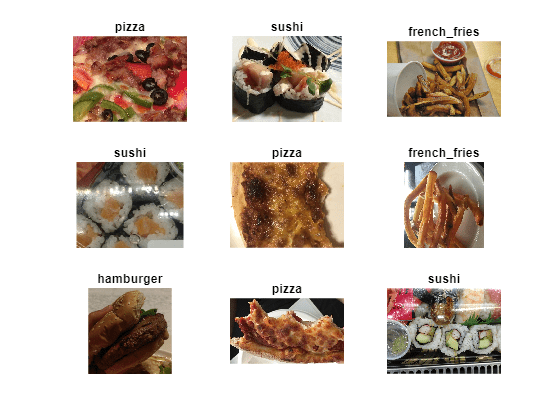### Train Network to Classify Food Images

Use the pretrained GoogLeNet network and train it again to classify the 9 types of food. If you don't have the Deep Learning Toolbox™ Model for GoogLeNet Network support package installed, then the software provides a download link.

To try a different pretrained network, open this example in MATLAB® and select a different network, such as `squeezenet`, a network that is even faster than `googlenet`. For a list of all available networks, see Pretrained Deep Neural Networks.

`net = googlenet;`

The first element of the `Layers` property of the network is the image input layer. This layer requires input images of size 224-by-224-by-3, where 3 is the number of color channels.

`inputSize = net.Layers(1).InputSize;`

#### Network Architecture

The convolutional layers of the network extract image features that the last learnable layer and the final classification layer use to classify the input image. These two layers, `'loss3-classifier'` and `'output'` in GoogLeNet, contain information on how to combine the features that the network extracts into class probabilities, a loss value, and predicted labels. To train a pretrained network to classify new images, replace these two layers with new layers adapted to the new data set.

Extract the layer graph from the trained network.

`lgraph = layerGraph(net);`

In most networks, the last layer with learnable weights is a fully connected layer. Replace this fully connected layer with a new fully connected layer with the number of outputs equal to the number of classes in the new data set (9, in this example).

```numClasses = numel(categories(imdsTrain.Labels)); newfclayer = fullyConnectedLayer(numClasses,... 'Name','new_fc',... 'WeightLearnRateFactor',10,... 'BiasLearnRateFactor',10); lgraph = replaceLayer(lgraph,net.Layers(end-2).Name,newfclayer);```

The classification layer specifies the output classes of the network. Replace the classification layer with a new one without class labels. `trainNetwork` automatically sets the output classes of the layer at training time.

```newclasslayer = classificationLayer('Name','new_classoutput'); lgraph = replaceLayer(lgraph,net.Layers(end).Name,newclasslayer);```

#### Train Network

The network requires input images of size 224-by-224-by-3, but the images in the image datastore have different sizes. Use an augmented image datastore to automatically resize the training images. Specify additional augmentation operations to perform on the training images: randomly flip the training images along the vertical axis, randomly translate them up to 30 pixels, and scale them up to 10% horizontally and vertically. Data augmentation helps prevent the network from overfitting and memorizing the exact details of the training images.

```pixelRange = [-30 30]; scaleRange = [0.9 1.1]; imageAugmenter = imageDataAugmenter( ... 'RandXReflection',true, ... 'RandXTranslation',pixelRange, ... 'RandYTranslation',pixelRange, ... 'RandXScale',scaleRange, ... 'RandYScale',scaleRange); augimdsTrain = augmentedImageDatastore(inputSize(1:2),imdsTrain, ... 'DataAugmentation',imageAugmenter);```

To automatically resize the validation images without performing further data augmentation, use an augmented image datastore without specifying any additional preprocessing operations.

`augimdsValidation = augmentedImageDatastore(inputSize(1:2),imdsValidation);`

Specify the training options. Set `InitialLearnRate` to a small value to slow down learning in the transferred layers that are not already frozen. In the previous step, you increased the learning rate factors for the last learnable layer to speed up learning in the new final layers. This combination of learning rate settings results in fast learning in the new layers, slower learning in the middle layers, and no learning in the earlier, frozen layers.

Specify the number of epochs to train for. When performing transfer learning, you do not need to train for as many epochs. An epoch is a full training cycle on the entire training data set. Specify the mini-batch size and validation data. Compute the validation accuracy once per epoch.

```miniBatchSize = 10; valFrequency = floor(numel(augimdsTrain.Files)/miniBatchSize); options = trainingOptions('sgdm', ... 'MiniBatchSize',miniBatchSize, ... 'MaxEpochs',4, ... 'InitialLearnRate',3e-4, ... 'Shuffle','every-epoch', ... 'ValidationData',augimdsValidation, ... 'ValidationFrequency',valFrequency, ... 'Verbose',false, ... 'Plots','training-progress');```

Train the network using the training data. By default, `trainNetwork` uses a GPU if one is available. This requires Parallel Computing Toolbox™ and a supported GPU device. For information on supported devices, see GPU Support by Release (Parallel Computing Toolbox). Otherwise, `trainNetwork` uses a CPU. You can also specify the execution environment by using the `'ExecutionEnvironment'` name-value pair argument of `trainingOptions`. Because this data set is small, the training is fast. If you run this example and train the network yourself, you will get different results and misclassifications caused by the randomness involved in the training process.

`net = trainNetwork(augimdsTrain,lgraph,options);`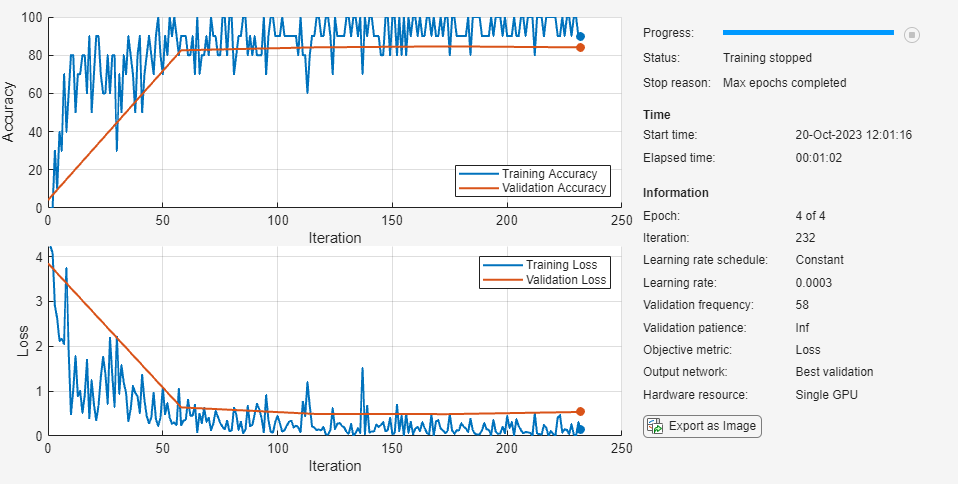#### Classify Test Images

Classify the test images using the fine-tuned network and calculate the classification accuracy.

```augimdsTest = augmentedImageDatastore(inputSize(1:2),imdsTest); [predictedClasses,predictedScores] = classify(net,augimdsTest); accuracy = mean(predictedClasses == imdsTest.Labels)```
```accuracy = 0.8418 ```

#### Confusion Matrix for the Test Set

Plot a confusion matrix of the test set predictions. This highlights which particular classes cause most problems for the network.

```figure; confusionchart(imdsTest.Labels,predictedClasses,'Normalization',"row-normalized");```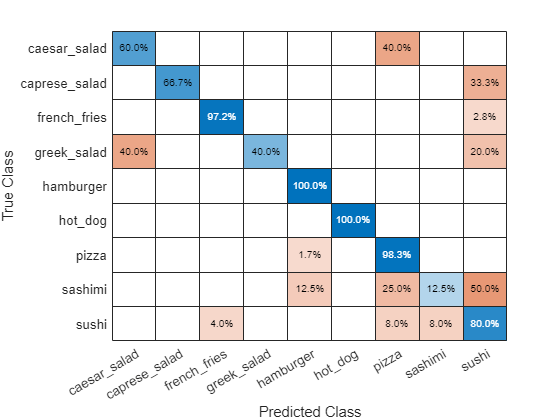The confusion matrix shows that the network has poor performance for some classes, such as Greek salad, sashimi, hot dog, and sushi. These classes are underrepresented in the data set which may be impacting network performance. Investigate one of these classes to better understand why the network is struggling.

```figure(); histogram(imdsValidation.Labels); ax = gca(); ax.XAxis.TickLabelInterpreter = "none";```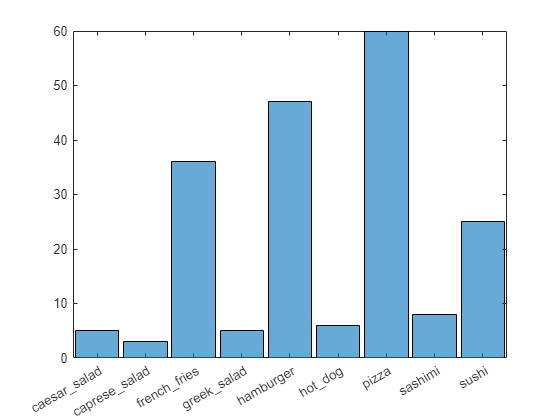### Investigate Classifications

Investigate network classification for the sushi class.

#### Sushi Most Like Sushi

First, find which images of sushi most strongly activate the network for the sushi class. This answers the question "Which images does the network think are most sushi-like?".

Plot the maximally-activating images, these are the input images that strongly activate the fully-connected layer's "sushi" neuron. This figure shows the top 4 images, in a descending class score.

```chosenClass = "sushi"; classIdx = find(net.Layers(end).Classes == chosenClass); numImgsToShow = 4; [sortedScores,imgIdx] = findMaxActivatingImages(imdsTest,chosenClass,predictedScores,numImgsToShow); figure plotImages(imdsTest,imgIdx,sortedScores,predictedClasses,numImgsToShow)```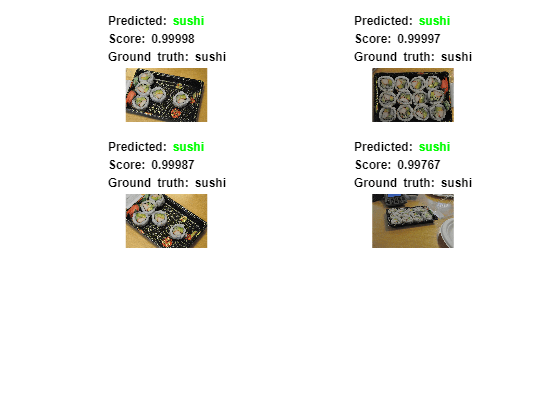#### Visualize Cues for the Sushi Class

Is the network looking at the right thing for sushi? The maximally-activating images of the sushi class for the network all look similar to each other - a lot of round shapes clustered closely together.

The network is doing well at classifying those kinds of sushi. However, to verify that this is true and to better understand why the network makes its decisions, use a visualization technique like Grad-CAM. For more information on using Grad-CAM, see Grad-CAM Reveals the Why Behind Deep Learning Decisions.

Read the first resized image from the augmented image datastore, then plot the Grad-CAM visualization using `gradCAM`.

```imageNumber = 1; observation = augimdsTest.readByIndex(imgIdx(imageNumber)); img = observation.input{1}; label = predictedClasses(imgIdx(imageNumber)); score = sortedScores(imageNumber); gradcamMap = gradCAM(net,img,label); figure alpha = 0.5; plotGradCAM(img,gradcamMap,alpha); sgtitle(string(label)+" (score: "+ max(score)+")")```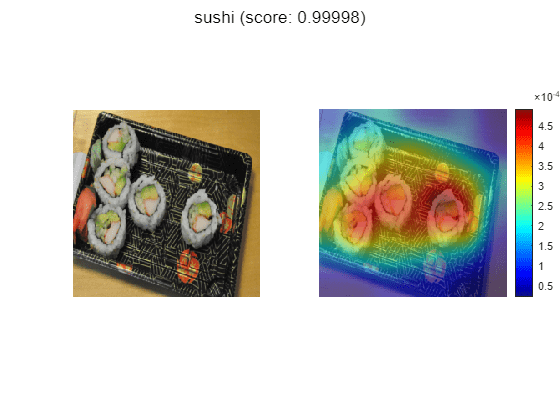The Grad-CAM map confirms that the network is focussing on the sushi in the image. However you can also see that the network is looking at parts of the plate and the table.

The second image has a cluster of sushi on the left and a lone sushi on the right. To see what the network focuses on, read the second image and plot the Grad-CAM.

```imageNumber = 2; observation = augimdsTest.readByIndex(imgIdx(imageNumber)); img = observation.input{1}; label = predictedClasses(imgIdx(imageNumber)); score = sortedScores(imageNumber); gradcamMap = gradCAM(net,img,label); figure plotGradCAM(img,gradcamMap,alpha); sgtitle(string(label)+" (score: "+ max(score)+")")```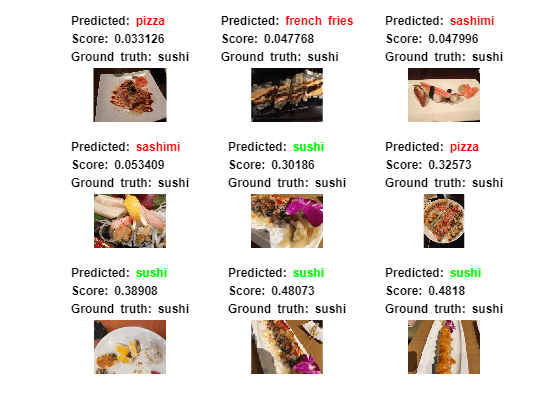The network classifies this image as sushi because it sees a group of sushi. However, is it able to classify one sushi on its own? Test this by looking at a picture of just one sushi.

```img = imread(strcat(tempdir,"Food Dataset/sushi/sushi_18.jpg")); img = imresize(img,net.Layers(1).InputSize(1:2),"Method","bilinear","AntiAliasing",true); [label,score] = classify(net,img); gradcamMap = gradCAM(net,img,label); figure alpha = 0.5; plotGradCAM(img,gradcamMap,alpha); sgtitle(string(label)+" (score: "+ max(score)+")")```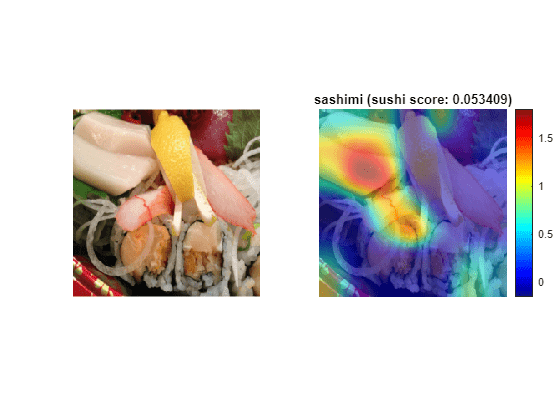The network is able to classify this lone sushi correctly. However, the GradCAM shows that the network is focussing on the top of the sushi and the cluster of cucumber, rather than the whole piece together.

Run the Grad-CAM visualization technique on a lone sushi that does not contain any stacked small pieces of ingredients.

```img = imread("crop__sushi34-copy.jpg"); img = imresize(img,net.Layers(1).InputSize(1:2),"Method","bilinear","AntiAliasing",true); [label,score] = classify(net,img); gradcamMap = gradCAM(net,img,label); figure alpha = 0.5; plotGradCAM(img,gradcamMap,alpha); title(string(label)+" (score: "+ max(score)+")")```In this case, the visualization technique highlights why the network performs poorly. It incorrectly classifies the image of the sushi as a hamburger.

To solve this issue, you must feed the network with more images of lone sushi during the training process.

#### Sushi Least Like Sushi

Now find which images of sushi activate the network for the sushi class the least. This answers the question "Which images does the network think are less sushi-like?".

This is useful because it finds the images on which the network performs badly, and it provides some insight into its decision.

```chosenClass = "sushi"; numImgsToShow = 9; [sortedScores,imgIdx] = findMinActivatingImages(imdsTest,chosenClass,predictedScores,numImgsToShow); figure plotImages(imdsTest,imgIdx,sortedScores,predictedClasses,numImgsToShow)```#### Investigate Sushi Misclassified as Sashimi

Why is the network classifying sushi as sashimi? The network classifies 3 out of the 9 images as sashimi. Some of these images, for example images 4 and 9, actually contain sashimi, which means the network isn't actually misclassifying them. These images are mislabeled.

To see what the network is focusing on, run the Grad-CAM technique on one of these images.

```imageNumber = 4; observation = augimdsTest.readByIndex(imgIdx(imageNumber)); img = observation.input{1}; label = predictedClasses(imgIdx(imageNumber)); score = sortedScores(imageNumber); gradcamMap = gradCAM(net,img,label); figure alpha = 0.5; plotGradCAM(img,gradcamMap,alpha); title(string(label)+" (sushi score: "+ max(score)+")")```As expected, the network focuses on the sashimi instead of the sushi.

#### Investigate Sushi Misclassified as Pizza

Why is the network classifying sushi as pizza? The network classifies four of the images as pizza instead of sushi. Consider image 1, this image has a colourful topping which may be confusing the network.

To see which part of the image the network is looking at, run the Grad-CAM technique on one of these images.

```imageNumber = 1; observation = augimdsTest.readByIndex(imgIdx(imageNumber)); img = observation.input{1}; label = predictedClasses(imgIdx(imageNumber)); score = sortedScores(imageNumber); gradcamMap = gradCAM(net,img,label); figure alpha = 0.5; plotGradCAM(img,gradcamMap,alpha); title(string(label)+" (sushi score: "+ max(score)+")")```The network strongly focuses on the toppings. To help the network distinguish pizza from sushi with toppings, add more training images of sushi with toppings. The network also focuses on the plate. This may be as the network has learned to associate certain foods with certain types of plates, as also highlighted when looking at the sushi images. To improve the network's performance, train using more examples of food on different types of plates.

#### Investigate Sushi Misclassified as a Hamburger

Why is the network classifying sushi as a hamburger? To see what the network is focusing on, run the Grad-CAM technique on the misclassified image.

```imageNumber = 2; observation = augimdsTest.readByIndex(imgIdx(imageNumber)); img = observation.input{1}; label = predictedClasses(imgIdx(imageNumber)); score = sortedScores(imageNumber); gradcamMap = gradCAM(net,img,label); figure alpha = 0.5; plotGradCAM(img,gradcamMap,alpha); title(string(label)+" (sushi score: "+ max(score)+")")```The network is focussing on the flower in the image. The colourful purple flower and brown stalk has confused the network into identifying this image as a hamburger.

#### Investigate Sushi Misclassified as French Fries

Why is the network classifying sushi as French fries? The network classifies the 3rd image as French fries instead of sushi. This specific sushi has a yellow topping and the network might associate this color with French fries.

```imageNumber = 3; observation = augimdsTest.readByIndex(imgIdx(imageNumber)); img = observation.input{1}; label = predictedClasses(imgIdx(imageNumber)); score = sortedScores(imageNumber); gradcamMap = gradCAM(net,img,label); figure alpha = 0.5; plotGradCAM(img,gradcamMap,alpha); title(string(label)+" (sushi score: "+ max(score)+")","Interpreter","none")```The networks focuses on the yellow sushi classifying it as French fries. As with the hamburger, the unusual colour has caused the network to misclassify the sushi.

To help the network in this specific case, train it with more images of yellow foods that are not French fries.

### Conclusions

Investigating the datapoints that give rise to large or small class scores, and datapoints that the network classifies confidently but incorrectly, is a simple technique which can provide useful insight into how a trained network is functioning. In the case of the food data set, this example highlighted that:

• The test data contains several images with incorrect true labels, such as the "sashimi" which is actually "sushi". The data also contains incomplete labels, such as images which contain both sushi and sashimi.

• The network considers a "sushi" to be "multiple, clustered, round-shaped things". However, it must be able to distinguish a lone sushi as well.

• Any sushi or sashimi with toppings or unusual colors confuses the network. To resolve this problem, the data must have a wider variety of sushi and sashimi.

• To improve performance the network needs to see more images from the underrepresented classes.

### Helper Functions

```function downloadExampleFoodImagesData(url,dataDir) % Download the Example Food Image data set, containing 978 images of % different types of food split into 9 classes. % Copyright 2019 The MathWorks, Inc. fileName = "ExampleFoodImageDataset.zip"; fileFullPath = fullfile(dataDir,fileName); % Download the .zip file into a temporary directory. if ~exist(fileFullPath,"file") fprintf("Downloading MathWorks Example Food Image dataset...\n"); fprintf("This can take several minutes to download...\n"); websave(fileFullPath,url); fprintf("Download finished...\n"); else fprintf("Skipping download, file already exists...\n"); end % Unzip the file. % % Check if the file has already been unzipped by checking for the presence % of one of the class directories. exampleFolderFullPath = fullfile(dataDir,"pizza"); if ~exist(exampleFolderFullPath,"dir") fprintf("Unzipping file...\n"); unzip(fileFullPath,dataDir); fprintf("Unzipping finished...\n"); else fprintf("Skipping unzipping, file already unzipped...\n"); end fprintf("Done.\n"); end function [sortedScores,imgIdx] = findMaxActivatingImages(imds,className,predictedScores,numImgsToShow) % Find the predicted scores of the chosen class on all the images of the chosen class % (e.g. predicted scores for sushi on all the images of sushi) [scoresForChosenClass,imgsOfClassIdxs] = findScoresForChosenClass(imds,className,predictedScores); % Sort the scores in descending order [sortedScores,idx] = sort(scoresForChosenClass,'descend'); % Return the indices of only the first few imgIdx = imgsOfClassIdxs(idx(1:numImgsToShow)); end function [sortedScores,imgIdx] = findMinActivatingImages(imds,className,predictedScores,numImgsToShow) % Find the predicted scores of the chosen class on all the images of the chosen class % (e.g. predicted scores for sushi on all the images of sushi) [scoresForChosenClass,imgsOfClassIdxs] = findScoresForChosenClass(imds,className,predictedScores); % Sort the scores in ascending order [sortedScores,idx] = sort(scoresForChosenClass,'ascend'); % Return the indices of only the first few imgIdx = imgsOfClassIdxs(idx(1:numImgsToShow)); end function [scoresForChosenClass,imgsOfClassIdxs] = findScoresForChosenClass(imds,className,predictedScores) % Find the index of className (e.g. "sushi" is the 9th class) uniqueClasses = unique(imds.Labels); chosenClassIdx = string(uniqueClasses) == className; % Find the indices in imageDatastore that are images of label "className" % (e.g. find all images of class sushi) imgsOfClassIdxs = find(imds.Labels == className); % Find the predicted scores of the chosen class on all the images of the % chosen class % (e.g. predicted scores for sushi on all the images of sushi) scoresForChosenClass = predictedScores(imgsOfClassIdxs,chosenClassIdx); end function plotImages(imds,imgIdx,sortedScores,predictedClasses,numImgsToShow) for i=1:numImgsToShow score = sortedScores(i); sortedImgIdx = imgIdx(i); predClass = predictedClasses(sortedImgIdx); correctClass = imds.Labels(sortedImgIdx); imgPath = imds.Files{sortedImgIdx}; if predClass == correctClass color = "\color{green}"; else color = "\color{red}"; end predClassTitle = strrep(string(predClass),'_',' '); correctClassTitle = strrep(string(correctClass),'_',' '); subplot(3,ceil(numImgsToShow./3),i) imshow(imread(imgPath)); title("Predicted: " + color + predClassTitle + "\newline\color{black}Score: " + num2str(score) + "\newlineGround truth: " + correctClassTitle); end end function plotGradCAM(img,gradcamMap,alpha) subplot(1,2,1) imshow(img); h = subplot(1,2,2); imshow(img) hold on; imagesc(gradcamMap,'AlphaData',alpha); originalSize2 = get(h,'Position'); colormap jet colorbar set(h,'Position',originalSize2); hold off; end```